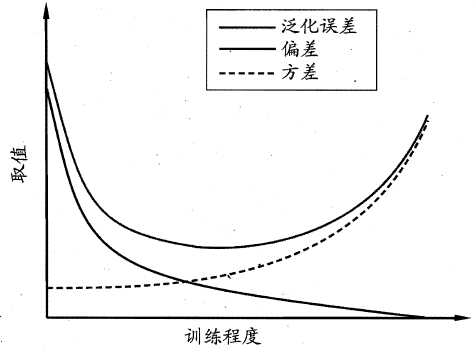# 《机器学习》周志华西瓜书学习笔记（二）：模型评估与选择

【机器学习】《机器学习》周志华西瓜书 笔记/习题答案 总目录

——————————————————————————————————————————————————————

# 模型评估与选择

## 误差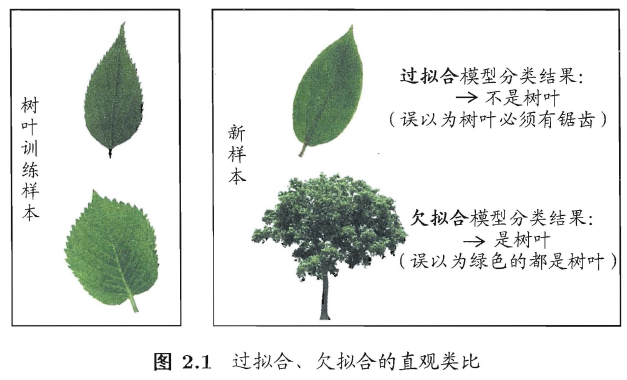P类问题：存在多项式时间算法的问题。
NP类问题：能在多项式时间内验证得出一个正确解的问题。
P类问题是NP问题的子集，因为存在多项式时间解法的问题，总能在多项式时间内验证它，但是存在多项式时间验证解的问题未必都能存在多项式时间算法。

• 评估方法：用什么数据做评估？如何获得这些数据？

• 性能度量：评估时如何衡量模型的好坏？有哪些评价标准？

• 比较检验：如何比较模型的性能？

——————————————————————————————————————————————————————

## 评估方法

Note！！！测试数据其实就是类比于模型在实际应用中遇到的数据，为了和模型评估中使用的测试数据进行区分，一般会把模型评估用的测试数据称为验证集（validation set）。举个例子，在Kaggle或者天池上参加比赛，我们一般会拿到一份带标记的原始数据集和一份不带标记的测试数据集。我们需要选用一种评估方法来把原始数据集划分成训练集和验证集，然后进行训练，并按照模型在验证集上的性能表现来进行选择，最后挑出最好的模型对测试集的样本进行预测，并提交预测结果。总结一下就是三个数据集：训练集、验证集（训练阶段的测试集）、测试集（最后用于测试模型性能的数据）。

#### 留出法

“留出法” (hold-out)直接将数据集$D$划分为两个互斥的集合，其中一个集合作为训练集$S$，另一个作为测试集$T$， 即$D=S \cup T, S \cap T=\varnothing$。在$S$上训练出模型后，用$T$来评估其测试误差，作为对泛化误差的估计。需注意的是，训练/测试集的划分要尽可能保持数据分布的一致性，避免因数据划分过程引入额外的偏差而对最终结果产生影响，例如在分类任务中至少要保持样本的类别比例相似。

$S$$T$ 中样本类别比例差别很大，则误差估计将由于训练/测试数据分布的差异而产生偏差。

#### 交叉验证

“交叉验证法” (cross validation)先将数据集$D$划分为$k$个大小相似的互斥子集， 即$D=D_{1} \cup D_{2} \cup \ldots \cup D_{k}, D_{i} \cap D_{j}=\varnothing(i \neq j)$。每个子集$D_i$都尽可能保持数据分布的一致性，即从$D$中通过分层采样得到。然后，每次用$k-1$个子集的并集作为训练集，余下的那个子集作为测试集；这样就可获得k组训练/测试集，从而可进行$k$次训练和测试，最终返回的是这$k$个测试结果的均值。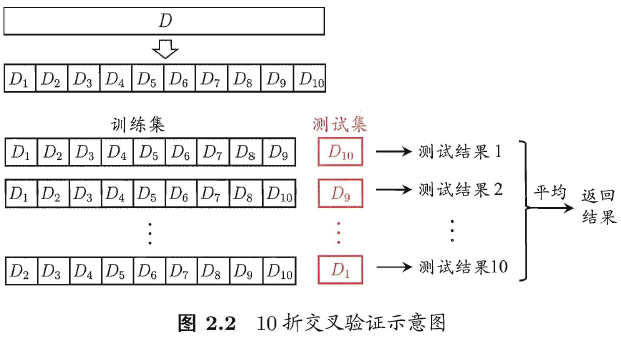10 次10 折交又验证法与100 次留出法都是进行了100 次训练/测试。

#### 自助法

Note！！！自助法适用于数据集小，难以划分训练/验证集的情况。此外，自助法能从初始数据集中产生多个不同的训练集，这对集成学习等方法有很大的好处。但是！自助法产生的数据集改变了初始数据集的分布，也因此引入了一些额外的误差。因此，在初始数据量足够时，留出法和交叉验证法更常用一些。

#### 调参和最终模型

——————————————————————————————————————————————————————

## 性能度量

#### 查准率，查全率，F1

$precision = \frac{TP}{TP+FP}$

$recall = \frac{TP}{TP+FN}$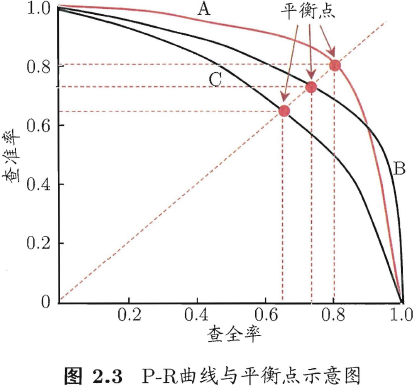F1，是查准率和查全率的调和平均，用于综合考虑这两个性能度量。

$\frac{1}{F1} = \frac{1}{2} \times (\frac{1}{precision} + \frac{1}{recall}) \Rightarrow F1 = \frac{2 \times precision \times recall}{presion + recall}$

$F_\beta$，是查准率和查全率的加权调和平均，用于综合考虑这两个性能度量，并采用不同的权重。

$\frac{1}{F_\beta} = \frac{1}{1+\beta^2} \times (\frac{1}{precision} + \frac{\beta^2}{recall}) \Rightarrow F_\beta = \frac{(1+\beta^2) \times precision \times recall}{(\beta^2 \times presion) + recall}$

#### ROC与AUC

$TPR = \frac{TP}{TP+FN}$

$FPR = \frac{FP}{TP+FN}$

TPR其实就等于召回率。在绘制ROC曲线时，纵轴为TPR，横轴为FPR。首先按预测值对样本进行排序，然后按序逐个把样本预测为正例，并计算此时的TPR和FPR，然后在图上画出该点，并与前一个点连线。如下图：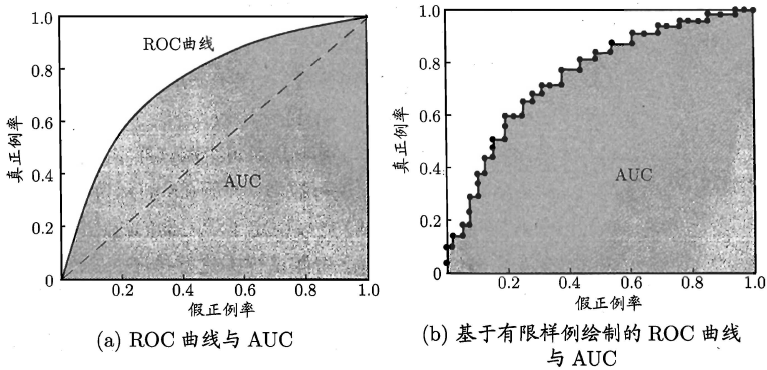• 经过 (0,1) 点的曲线，这代表所有正例都在反例之前出现（否则会先出现假正例从而无法经过 (0,1) 点），这是一个理想模型，我们可以设置一个阈值，完美地分割开正例和反例。

• 对角线，这对应于随机猜测模型，可以理解为真正例和假正例轮换出现，即每预测对一次接下来就预测错一次，可以看作是随机猜测的结果。

$AUC=\frac{1}{2}\sum_{i=1}^{m-1}(x_{i+1}-x_i)\cdot(y_i+y_{i+1})$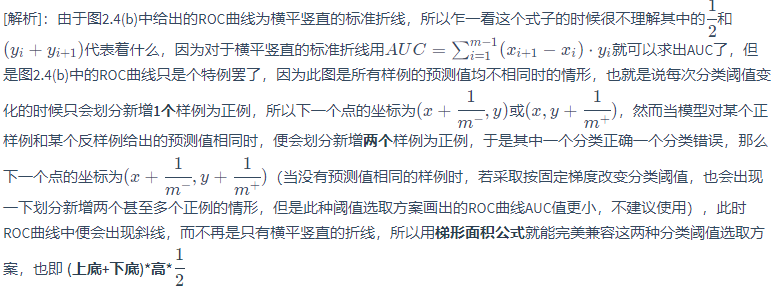$AUC = 1 - \ell_{rank}$

#### 代价敏感错误率与代价曲线

$E(f;D;cost) = \frac{1}{m}\lgroup\sum_{x_i \in D^+}\mathbb{I}(f(x_i) \neq y_i) \times cost_{01} + \sum_{x_i \in D^-}\mathbb{I}(f(x_i) \neq y_i) \times cost_{10}\rgroup$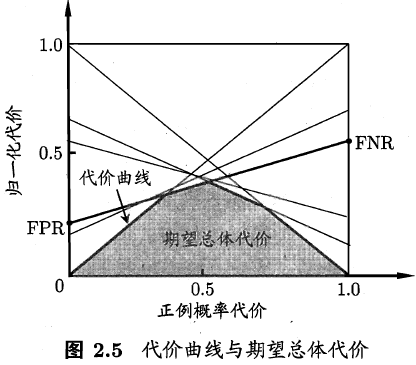——————————————————————————————————————————————————————

## 比较检验

Note！！！指验证集，但无论是书中还是论文中，都使用测试集较多，明白两者的区别就可以了。

1. 希望比较的是泛化性能，然而通过实验评估方法我们获得的是测试集上的性能，两者的对比结果可能未必相同；
2. 测试集上的性能与测试集本身的选择有很大关系，且不论使用不同大小的测试集会得到不同的结果，即使用相同大小的测试集，若包含的测试样例不同，测试结果也会有不同；
3. ，很多机器学习算法本身有一定的随机性，即便用相同的参数设置在同一个测试集上多次运行，其结果也会有不同；

• 置信度：表示有多大的把握认为假设是正确的。
• 显著度：也称“显著性水平”，表示假设出错的概率。显著度越大，假设被拒绝的可能性越大。
• 自由度：不被限制的样本数，也可以理解为能自由取值的样本数，记为 $v$$df$

### 单个模型、单个数据集上的泛化性能检验

#### 二项检验

$P(\hat{\epsilon};\epsilon) = \binom{m}{m'} \epsilon^{m'} (1-\epsilon)^{m - m'}$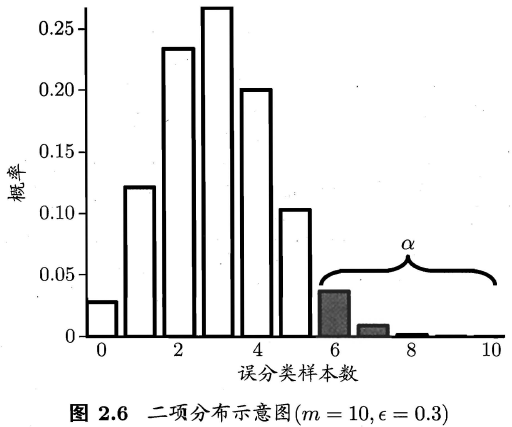α 的常用取位有0.05 、0.1 ，图2.6 中α 较大是为了绘图方便。

$\overline{\epsilon} = \max{\epsilon} \qquad s.t. \qquad \sum_{i=\epsilon_0 \times m+1}^m \binom{m}{i}\epsilon^i (1-\epsilon)^{m-i} < \alpha$

#### t检验

$\mu = \frac{1}{k} \sum_{i=1}^k \hat{\epsilon_i}$

$\sigma^2 = \frac{1}{k-1} \sum_{i=1}^k (\hat{\epsilon_i} - \mu)^2$

$t = \frac{\sqrt{k}(\mu-\epsilon_0)}{\sigma}$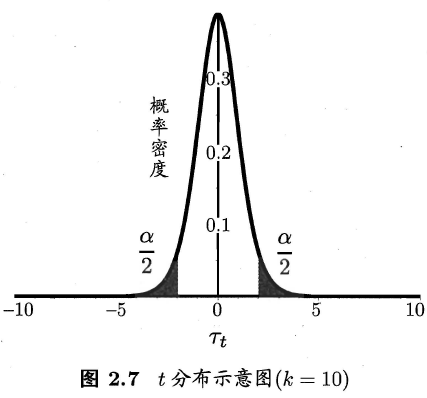### 两个模型/算法、单个数据集上的泛化性能检验

#### 交叉验证t检验

$t = \lvert \frac{\sqrt{k}\mu}{\sigma} \rvert$

#### McNemar检验

$\chi^2 = \frac{(\lvert e_{01}-e_{10} \rvert -1)^2}{e_{01} + e_{10}}$

### 多个模型/算法、多个数据集上的泛化性能检验

#### Friedman检验

D1 1 2 3
D2 1 2.5 2.5
D3 1 2 3
D4 1 2 3

$r_i$ 表示第 $i$ 个算法的平均序值，则 $r_i$ 服从均值为 $\frac{k+1}{2}$，方差为 $\frac{(k^2)-1}{12}$ 的正态分布。可以计算统计量 $\chi^2$

$\chi^2 = \frac{12N}{k(k+1)}(\sum_{i=1}^k r_i^2 - \frac{k(k+1)^2}{4})$

$k$$N$ 都较大时(通常要求 $k>30$)，该变量服从自由度为 $v=k-1$$\chi^2$ 分布（卡方分布）。

$F = \frac{(N-1)\chi^2}{N(k-1)-\chi^2}$

#### Nemenyi后续检验

$CD = q_\alpha \sqrt{\frac{k(k+1)}{6N}}$

Nemenyi后续检验还可以通过Friedman检验图更直观地体现出来，横轴为性能度量，纵轴为算法，每个算法用一段水平线段表示，线段中心点为该算法的平均序值，线段长度为 $CD$。若两个算法的线段投影到x轴上有重叠部分，则可以认为这两个算法的泛化性能无显著区别。

## 偏差与方差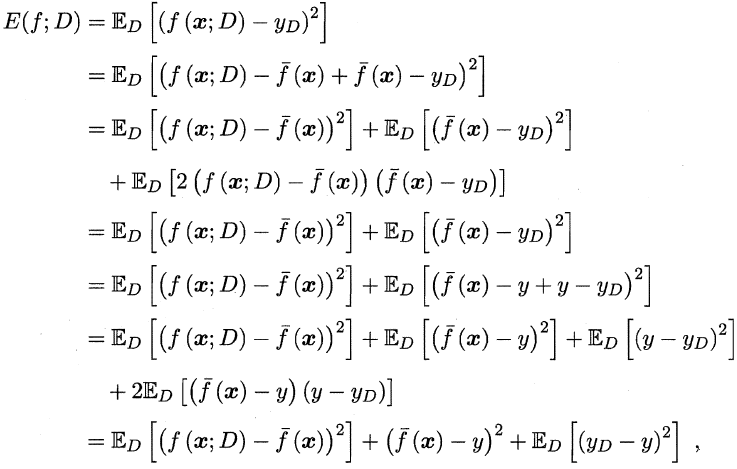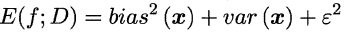• 方差：度量了同样大小的训练集的变动所导致的学习性能的变化，刻画数据扰动所造成的影响

• 偏差：度量了学习算法的期望预测与真实结果的偏离程度，刻画学习算法本身的拟合能力

• 噪声：表达了在当前任务上任何学习算法所能达到的期望泛化误差的下界，刻画学习问题本身的难度